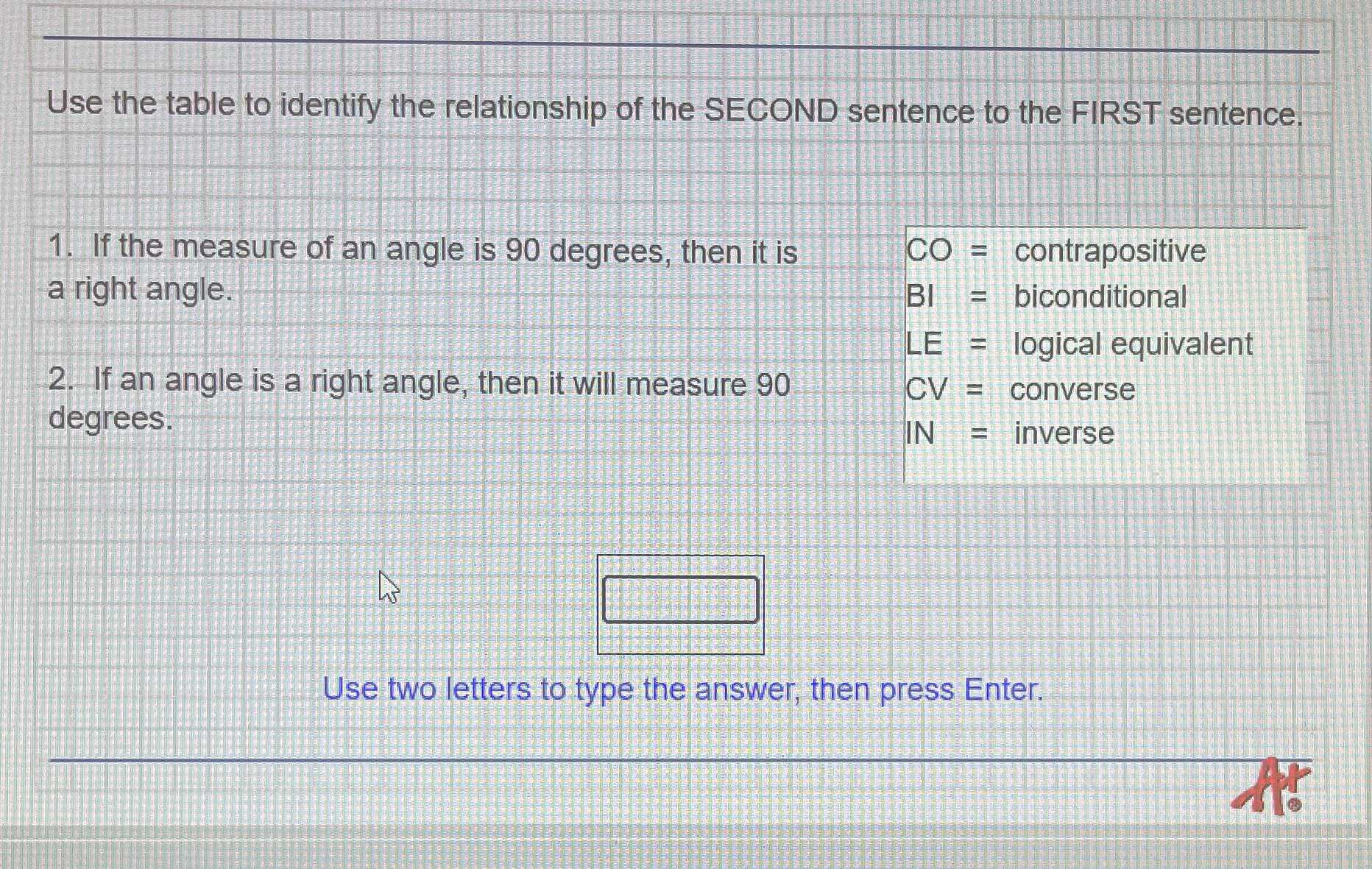### Still have math questions?

Trigonometry
QuestionUse the table to identify the relationship of the SECOND sentence to the FIRST sentence.

CO = contrapositive

$$BI =$$ contrapositive

$$LE =$$ biconditional

$$CV =$$ converse

IN $$=$$ inverse

1. If the measure of an angle is $$90$$ degrees, then it is a right angle.

2. If an angle is a right angle, then it will measure $$90$$ degrees.